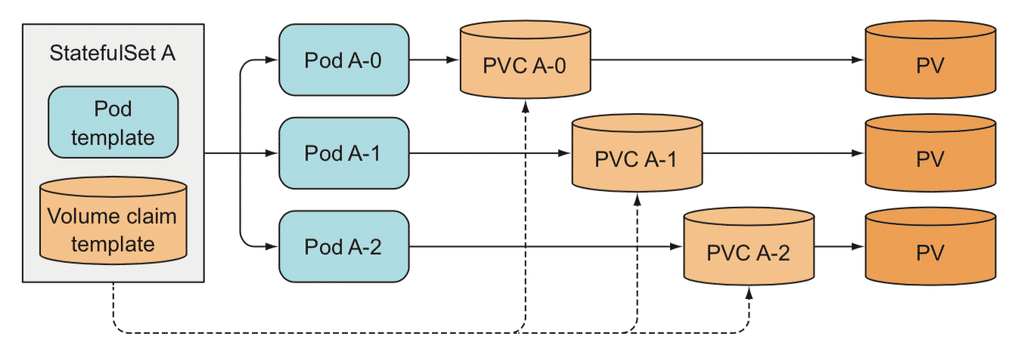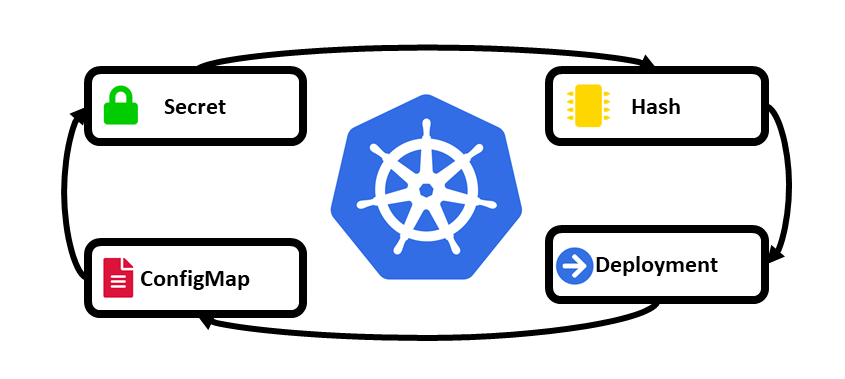Python 描述器

``````class A:
def __init__(self, name, score):
self.name = name # 普通属性
self._score = score

@property
def score(self):
return self._score

@score.setter
def score(self, value):
print('setting score here')
if isinstance(value, int):
self._score = value
else:

a = A('Bob',90)
a.name # 'Bob'
a.score # 90
a.name = 1
a.name # 1 ，名字本身不应该允许赋值为数字，但是这里无法控制其赋值
a.score = 83
a.score # 83，当赋值为数值型的时候，可以顺利运行
a.score = 'bob' # please input an int
a.score # 83，赋值为字符串时，score没有被改变
``````

``````class A:
def __init__(self, name, score, age):
self.name = name # 普通属性
self._score = score
self._age = age

@property
def score(self):
return self._score

@score.setter
def score(self, value):
print('setting score here')
if isinstance(value, int):
self._score = value
else:

@property
def age(self):
return self._age

@age.setter
def age(self, value):
print('setting age here')
if isinstance(value, int):
self._age = value
else:

a = A('Bob', 90, 20)
``````

## 描述器基本理论及简单实例

``````# 创建一个描述器的类，它的实例就是一个描述器
# 这个类要有__get__  __set__ 这样的方法
# 这种类是当做工具使用的，不单独使用
class M:
def __init__(self, x=1):
self.x = x

def __get__(self, instance, owner):
return self.x

def __set__(self, instance, value):
self.x = value

# 调用描述器的类
class AA:
m = M() # m就是一个描述器

aa = AA()
aa.m # 1
aa.m = 2
aa.m # 2
``````

• 创建aa实例和普通类没什么区别，我们从`aa.m`开始看
• `aa.m`是aa实例调用了m这个类属性，然而这个类属性不是普通的值，而是一个描述器，所以我们从访问这个类属性变成了访问这个描述器
• 如果调用时得到的是一个描述器，python内部就会自动触发一套使用机制
• 访问的话自动触发描述器的`__get__`方法
• 修改设置的话就自动触发描述器的`__set__`方法
• 这里就是`aa.m`触发了`__get__`方法，得到的是`self.x`的值，在前面`__init__`中定义的为1
• `aa.m = 2`则触发了`__set__`方法，赋的值2传到`value`参数之中，改变了`self.x`的值，所以下一次`aa.m`调用的值也改变了

## 描述器的调用机制

`aa.m`命令其实是查找m属性的过程，程序会先到哪里找，没有的话再到哪里找，这是有一个顺序的，说明访问顺序时需要用到`__dict__`方法。

``````class C:
x = 1
def __init__(self, y):
self.y = y

def fun(self):
print(self.y)

c = C(2)
# 实例有哪些属性
print(c.__dict__) # {'y': 2}
# 类有什么属性
print(C.__dict__) # 里面有 x fun
print(type(c).__dict__) # 和上一条一样

print(vars(c)) # __dict__ 也可以用 vars 函数替代，功能完全相同

# 调用
c.fun() # 2
c.__dict__['y'] # 2
# type(c).__dict__['fun']() # 报错，说明函数不是这么调用的
``````

`__dict__`方法返回的是一个字典，类和实例都可以调用，键就是类或实例所拥有的属性、方法，可以用这个字典访问属性，但是方法就不能这样直接访问，原因我们之后再说。

• 程序会先查找 `aa.__dict__['m']` 是否存在
• 不存在再到`type(aa).__dict__['m']`中查找
• 然后找`type(aa)`的父类
• 期间找到的是普通值就输出，如果找到的是一个描述器，则调用`__get__`方法

``````class M:
def __init__(self):
self.x = 1

def __get__(self, instance, owner):
return self.x

def __set__(self, instance, value):
self.x = value

# 调用描述器的类
class AA:
m = M() # m就是一个描述器
n = 2
def __init__(self, score):
self.score = score

aa = AA(3)
print(aa.__dict__) # {'score': 3}
print(aa.score) # 3, 在 aa.__dict__ 中寻找，找到了score直接返回
print(aa.__dict__['score']) # 3, 上面的调用机制实际上是这样的

print(type(aa).__dict__) # 里面有n和m
print(aa.n) # 2, 在aa.__dict__中找不到n，于是到type(aa).__dict__中找到了n，并返回其值
print(type(aa).__dict__['n']) # 2, 其实是上面一条的调用机制

print(aa.m) # 1, 在aa.__dict__中找不到n，于是到type(aa).__dict__中找到了m
# m是一个描述器对象，于是调用__get__方法，将self.x的值返回，即1
print(type(aa).__dict__['m'].__get__(aa,AA)) # 1, 上面一条的调用方式是这样的
# __get__的定义中，除了self，还有instance和owner，其实分别表示的就是描述器所在的实例和类，这里的细节我们后文会讲

print('-'*20)
print(AA.m) # 1, 也是一样调用了描述器
print(AA.__dict__['m'].__get__(None, AA)) # 类相当于调用这个
``````

• 同时定义了`__get__``__set__`方法的描述器称为资料描述器
• 只定义了`__get__`的描述器称为非资料描述器
• 二者的区别是：当属性名和描述器名相同时，在访问这个同名属性时，如果是资料描述器就会先访问描述器，如果是非资料描述器就会先访问属性 举例如下
``````# 既有__get__又有__set__，是一个资料描述器
class M:
def __init__(self):
self.x = 1

def __get__(self, instance, owner):
print('get m here') # 打印一些信息，看这个方法何时被调用
return self.x

def __set__(self, instance, value):
print('set m here') # 打印一些信息，看这个方法何时被调用
self.x = value + 1 # 这里设置一个+1来更清楚了解调用机制

# 只有__get__是一个非资料描述器
class N:
def __init__(self):
self.x = 1

def __get__(self, instance, owner):
print('get n here') # 打印一些信息，看这个方法何时被调用
return self.x

# 调用描述器的类
class AA:
m = M() # m就是一个描述器
n = N()
def __init__(self, m, n):
self.m = m # 属性m和描述器m名字相同，调用时发生一些冲突
self.n = n # 非资料描述器的情况，与m对比

aa = AA(2,5)
print(aa.__dict__) # 只有n没有m, 因为资料描述器同名时，不会访问到属性，会直接访问描述器，所以属性里就查不到m这个属性了
print(AA.__dict__) # m和n都有
print(aa.n) # 5, 非资料描述器同名时调用的是属性，为传入的5
print(AA.n) # 1, 如果是类来访问，就调用的是描述器，返回self.x的值

print(aa.m) # 3, 其实在aa=AA(2,5)创建实例时，进行了属性赋值，其中相当于进行了aa.m=2
# 但是aa调用m时却不是常规地调用属性m，而是资料描述器m
# 所以定义实例aa时，其实触发了m的__set__方法，将2传给value，self.x变成3
# aa.m调用时也访问的是描述器，返回self.x即3的结果
# 其实看打印信息也能看出什么时候调用了__get__和__set__

aa.m = 6 # 另外对属性赋值也是调用了m的__set__方法
print(aa.m) # 7，调用__get__方法

print('-'*20)
# 在代码中显式调用__get__方法
print(AA.__dict__['n'].__get__(None, AA)) # 1
print(AA.__dict__['n'].__get__(aa, AA)) # 1
``````

## 描述器的细节

• 调用描述器的原理
• `__get__``__set__`方法中的参数解释

1.首先是调用描述器的原理 当调用一个属性，而属性指向一个描述器时，为什么就会去调用这个描述器呢，其实这是由`object.__getattribute__()`方法控制的，其中`object`是新式类定义时默认继承的类，即py2这么写的`class(object)`中的`object`。新定义的一个类继承了object类，也就继承了`__getattribute__`方法。当访问一个属性比如`b.x`时，会自动调用这个方法 `__getattribute__()`的定义如下

``````def __getattribute__(self, key):
"Emulate type_getattro() in Objects/typeobject.c"
v = object.__getattribute__(self, key)
if hasattr(v, '__get__'):
return v.__get__(None, self)
return v
``````

2.`__get__``__set__`方法中的参数解释 官网中标明了这三个方法需要传入哪些参数，还有这些方法的返回结果是什么，如下所示

``````descr.__get__(self, obj, type=None) --> value
descr.__set__(self, obj, value) --> None
descr.__delete__(self, obj) --> None
``````

``````class M:
def __init__(self, name):
self.name = name

def __get__(self, obj, type):
print('get第一个参数self: ', self.name)
print('get第二个参数obj: ', obj.age)
print('get第三个参数type: ', type.name)

def __set__(self, obj, value):
obj.__dict__[self.name] = value

class A:
name = 'Bob'
m = M('age')
def __init__(self, age):
self.age = age

a = A(20) # age是20
a.m
# get第一个参数self:  age
# get第二个参数obj:  20
# get第三个参数type:  Bob
a.m = 30
a.age # 30
``````

• self是描述器类M中的实例
• obj是调用描述器的类a中的实例
• type是调用描述器的类A
• value是对这个属性赋值时传入的值，即上面的30

• `a.m`访问描述器，调用`__get__`方法
• 三次打印分别调用了`m.name a.age A.name`
• `a.m = 30`调用了`__set__`方法，令a(即obj)的属性中的’age’(即M(‘age’)这里传入的self.name)为30

## 实例方法、静态方法和类方法的描述器原理

``````class B:
@classmethod
def print_classname(cls):
print('Bob')

@staticmethod
def print_staticname():
print('my name is bob')

def print_name(self):
print('this name')

b = B()
b.print_classname() # 调用类方法
b.print_staticname() # 调用静态方法
b.print_name() # 调用实例方法
print(B.__dict__) # 里面有实例方法、静态方法和类方法
``````
``````# 但其实字典里的还不是可以直接调用的函数
print(B.__dict__['print_classname'])
print(b.print_classname) # 和上不一样
print(B.__dict__['print_staticname'])
print(b.print_staticname) # 和上不一样
print(B.__dict__['print_name'])
print(b.print_name) # 和上不一样

# <classmethod object at 0x0000024A92DA67B8>
# <bound method B.print_classname of <class '__main__.B'>>
# <staticmethod object at 0x0000024A92DA6860>
# <function B.print_staticname at 0x0000024A92D889D8>
# <function B.print_name at 0x0000024A92D88158>
# <bound method B.print_name of <__main__.B object at 0x0000024A92DA6828>>
``````

``````B.__dict__['print_classname'].__get__(None, B)()
B.__dict__['print_staticname'].__get__(None, B)()
B.__dict__['print_name'].__get__(b, B)()

print(B.__dict__['print_classname'].__get__(None, B))
print(B.__dict__['print_staticname'].__get__(None, B))

print(B.__dict__['print_name'])
print(B.__dict__['print_name'].__get__(None, B)) # 这是不传入实例即self的情况，和直接从字典调用结果相同，在python2中是一个unbound method
print(B.__dict__['print_name'].__get__(b, B))

# B.print_name() # 报错，说少输入一个self参数
# B.print_name(B()) # this name  输入实例即不会报错
``````

``````class myclassmethod(object):
def __init__(self, method):
self.method = method
def __get__(self, instance, cls):
return lambda *args, **kw: self.method(cls, *args, **kw)

class Myclass:
x = 3
@myclassmethod
def method(cls, a):
print(cls.x+a)

m = Myclass()
Myclass.method(a=2)
``````

• 我们看到使用@myclassmethod装饰器达到的效果和使用@classmethod装饰器没有什么区别
• 首先定义了myclassmethod类，里面使用了`__get__`方法，所以它的实例会是一个描述器对象
• `myclassmethod`当做装饰器作用于method函数，根据装饰器的知识，相当于这样设置`method=myclassmethod(method)`
• 调用`Myclass.method()`时调用了改变后了的`method`方法，即`myclassmethod(method)(a)`
• `myclassmethod(method)`这是`myclassmethod`类的一个实例，即一个描述器，此处访问于是调用`__get__`方法，返回一个匿名函数
• `__get__`中其实是将owner(cls)部分传入method方法，因为methon在Myclass类中调用，这个owner也就是Myclass类。这一步其实是提前传入了method的第一个参数cls，后面的参数a由`myclassmethod(method)(a)`第二个括号调用
• 仔细分析上面的定义与调用过程，我们会发现，我们常常说的类方法第一个参数要是cls，其实是不对的，第一个参数是任意都可以，它只是占第一个位置，用于接收类实例引用类属性，随便换成任意变量都可以，用cls只是约定俗成的。比如下面的代码正常运行
``````class Myclass:
x = 3
@classmethod
def method(b, a):
print(b.x+a)

m = Myclass()
Myclass.method(a=2) # 5
``````

``````class mystaticmethod:
def __init__(self, callable):
self.f = callable
def __get__(self, obj, type=None):
return self.f

class Myclass:
x = 3
@mystaticmethod
def method(a, b):
print(a + b)

m = Myclass()
m.method(a=2, b=3)
``````

• 静态方法相当于不自动传入实例对象作为方法的第一个参数，类方法相当于将默认传入的第一个参数由实例改为类
• 使用`@classmethod`后无论类调用还是实例调用，都会自动转入类作为第一个参数，不用手动传入就可以调用类属性，而没有`@classmethod`的需要手动传入类
• 既不用`@classmethod`也不用`@staticmethod`则类调用时不会自动传入参数，实例调用时自动传入实例作为第一个参数
• 所以说加`@classmethod`是为了更方便调用类属性，加`@staticmethod`是为了防止自动传入的实例的干扰
• 除此之外要说明一点：当属性和方法重名时，调用会自动访问属性，是因为这些方法调用的描述器都是非资料描述器。而当我们使用`@property`装饰器后，自动调用的就是新定义的`get set`方法，是因为`@property`装饰器是资料描述器

## property装饰器的原理

``````class Property(object):
"Emulate PyProperty_Type() in Objects/descrobject.c"

def __init__(self, fget=None, fset=None, fdel=None, doc=None):
self.fget = fget
self.fset = fset
self.fdel = fdel
if doc is None and fget is not None:
doc = fget.__doc__
self.__doc__ = doc

def __get__(self, obj, objtype=None):
if obj is None:
return self
if self.fget is None:
return self.fget(obj)

def __set__(self, obj, value):
if self.fset is None:
raise AttributeError("can't set attribute")
self.fset(obj, value)

def __delete__(self, obj):
if self.fdel is None:
raise AttributeError("can't delete attribute")
self.fdel(obj)

def getter(self, fget):
return type(self)(fget, self.fset, self.fdel, self.__doc__)

def setter(self, fset):
return type(self)(self.fget, fset, self.fdel, self.__doc__)

def deleter(self, fdel):
return type(self)(self.fget, self.fset, fdel, self.__doc__)
``````

``````# 类似函数的形式
class A:
def __init__(self, name, score):
self.name = name # 普通属性
self.score = score

def getscore(self):
return self._score

def setscore(self, value):
print('setting score here')
if isinstance(value, int):
self._score = value
else:

score = property(getscore, setscore)

a = A('Bob',90)
a.name # 'Bob'
a.score # 90
a.score = 'bob' # please input an int
``````

• 初始化时即开始访问score，发现有两个选项，一个是属性，另一个是`property(getscore, setscore)`对象，因为后者中定义了`__get__``__set__`方法，因此是一个资料描述器，具有比属性更高的优先级，所以这里就访问了描述器
• 因为初始化时是对属性进行设置，所以自动调用了描述器的`__set__`方法
• `__set__`中对`fset`属性进行检查，这里即传入的`setscore`，不是`None`，所以调用了`fset``setscore`方法，这就实现了设置属性时使用自定义函数进行检查的目的
• `__get__`也是一样，查询score时，调用`__get__`方法，触发了`getscore`方法

``````# 装饰器形式，即引言中的形式
class A:
def __init__(self, name, score):
self.name = name # 普通属性
self.score = score

@property
def score(self):
print('getting score here')
return self._score

@score.setter
def score(self, value):
print('setting score here')
if isinstance(value, int):
self._score = value
else:

a = A('Bob',90)
# a.name # 'Bob'
# a.score # 90
# a.score = 'bob' # please input an int
``````

• 在第一种使用方法中，是将函数作为传入property中，所以可以想到是否可以用装饰器来封装
• get部分很简单，访问`score`时，加上装饰器变成访问`property(score)`这个描述器，这个`score`也作为`fget`参数传入`__get__`中指定调用时的操作
• 而set部分就不行了，于是有了`setter`等方法的定义
• 使用了`property``setter`装饰器的两个方法的命名都还是score，一般同名的方法后面的会覆盖前面的，所以调用时调用的是后面的`setter`装饰器处理过的`score`，是以如果两个装饰器定义的位置调换，将无法进行属性赋值操作。
• 而调用`setter`装饰器的`score`时，面临一个问题，装饰器`score.setter`是什么呢？是`score``setter`方法，而`score`是什么呢，不是下面定义的这个`score`，因为那个`score`只相当于参数传入。自动向其他位置寻找有没有现成的`score`，发现了一个，是`property`修饰过的`score`，这是个描述器，根据`property`的定义，里面确实有一个`setter`方法，返回的是`property`类传入`fset`后的结果，还是一个描述器，这个描述器传入了`fget``fset`，这就是最新的`score`了，以后实例只要调用或修改`score`，使用的都是这个描述器
• 如果还有`del`则装饰器中的`score`找到的是`setter`处理过的`score`，最新的`score`就会是三个函数都传入的`score`
• 对最新的`score`的调用及赋值删除都跟前面一样了

`property`的原理就讲到这里，从它的定义我们可以知道它其实就是将我们设置的检查等函数传入`get set`等方法中，让我们可以自由对属性进行操作。它是一个框架，让我们可以方便传入其他操作，当很多对象都要进行相同操作的话，重复就是难免的。如果想要避免重复，只有自己写一个类似`property`的框架，这个框架不是传入我们希望的操作了，而是就把这些操作放在框架里面，这个框架因为只能实现一种操作而不具有普适性，但是却能大大减少当前问题代码重复问题

``````class Checkint:

def __init__(self, name):
self.name = name

def __get__(self, instance, owner):
if instance is None:
return self
else:
return instance.__dict__[self.name]

def __set__(self, instance, value):
if isinstance(value, int):
instance.__dict__[self.name] = value
else:

# 类似函数的形式
class A:
score = Checkint('score')
age = Checkint('age')

def __init__(self, name, score, age):
self.name = name # 普通属性
self.score = score
self.age = age

a = A('Bob', 90, 30)
a.name # 'Bob'
a.score # 90
# a.score = 'bob' # please input an int
# a.age='a' # please input an integer
``````

## 描述器的应用

• 首先是上文提到的，它是实例方法、静态方法、类方法、property的实现原理
• 当访问属性、赋值属性、删除属性，出现冗余操作，或者苦思无法找到答案时，可以求助于描述器
• 具体使用1：缓存。比如调用一个类的方法要计算比较长的时间，这个结果还会被其他方法反复使用，我们不想每次使用和这个相关的函数都要把这个方法重新运行一遍，于是可以设计出第一次计算后将结果缓存下来，以后调用都使用存下来的结果。只要使用描述器在`__get__`方法中，在判断语句下，`obj.__dict__[self.name] = value`。这样每次再调用这个方法都会从这个字典中取得值，而不是重新运行这个方法。

上一篇StatefulSet
StatefulSet 用于管理有状态 Pod 的控制器，例如 Redis，ZK 等，与 Deployment 相反。在 K8s 上定义和管理有状态的应用是比较困难的，每个有状态的服务都有自己的特性，你要考虑它们出现问题之后的重启方式，数据
2019-06-22K8s 存储卷、ConfigMap 和 Secret

2019-06-16
目录